Courses

# Test: 35 Year JEE Previous Year Questions: The d- and f-Block Elements & Coordination Compounds

## 74 Questions MCQ Test Class 12 Chemistry 35 Years JEE Mains &Advance Past yr Paper | Test: 35 Year JEE Previous Year Questions: The d- and f-Block Elements & Coordination Compounds

Description
This mock test of Test: 35 Year JEE Previous Year Questions: The d- and f-Block Elements & Coordination Compounds for JEE helps you for every JEE entrance exam. This contains 74 Multiple Choice Questions for JEE Test: 35 Year JEE Previous Year Questions: The d- and f-Block Elements & Coordination Compounds (mcq) to study with solutions a complete question bank. The solved questions answers in this Test: 35 Year JEE Previous Year Questions: The d- and f-Block Elements & Coordination Compounds quiz give you a good mix of easy questions and tough questions. JEE students definitely take this Test: 35 Year JEE Previous Year Questions: The d- and f-Block Elements & Coordination Compounds exercise for a better result in the exam. You can find other Test: 35 Year JEE Previous Year Questions: The d- and f-Block Elements & Coordination Compounds extra questions, long questions & short questions for JEE on EduRev as well by searching above.
QUESTION: 1

### A square planar complex is formed by hybridisation of which atomic orbitals?

Solution:

A square planar complex is formed by hybridisation of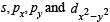atomic orbitals

QUESTION: 2

### The type of isomerism present in nitropentammine chromium (III) chloride is

Solution:

The  chemical formula of nitropentammine chromium (III) chloride is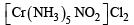It can exist in following two structures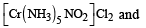nitropentammine chromium (III) chloride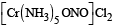Nitropentammine chromium (III) chloride Therefore the type of isomerism found in this compound is linkage isomerism as nitro groupis linked through N as –NO2 or through O as –ONO.

QUESTION: 3

### CH3 – Mg – Br is an organo metallic compound due to

Solution:

Compounds that contain at least one carbon metal bond are known as organometallic compounds. In CH3-Mg-Br (Grignard's reagent) a bond is present between carbon and Mg (Metal) hence it is an organometallic compound.

QUESTION: 4

Most common oxidation states of Ce (cerium) are

Solution:

Common oxidation states of Ce (Cerium) are + 3 and + 4

QUESTION: 5

Arrange Ce+3, La+3, Pm+3 and Yb+3 in increasing order of their ionic radii.

Solution:

In lanthanides there is a regular decrease in the atomic radii as well as ionic radii of trivalent ions as the atomic number increases from Ce to Lu. This decrease in size of atoms and  ions is known as lanthanide contraction.
Although the atomic radii do show some irregualrities but ionic radii decreases from La to Lu. Thus the correct order is.

Yb+3    <   Pm +3  <    Ce +3 < La +3
86.8pm   97pm     1 02pm  103pm

QUESTION: 6

Which of the following ions has the maximum magnetic moment?

Solution:

Mn++ –5 unpaired electrons
Fe++ – 4 unpaired electrons
Ti++ – 2 unpaired electrons
Cr++ – 4 unpaired electrons hence maximum no. of unpaired electron is present in Mn++.

NOTE : Magnetic moment × number of unpaired electrons

QUESTION: 7

The most stable ion is

Solution:

The cyano and hydroxo complexes are far more stable than those formed by halide ion. This is due to the fact that CN and OH are strong lewis bases (nucleophiles).
Further [Fe(OH)5]3– is not formed. hence most stable ion is [Fe(CN)6]3-

QUESTION: 8

When KMnO4 acts as an oxidising agent and ultimately forms [MnO4]–2, MnO2, Mn2O3, Mn+2 then the number of electrons transferred in each case respectively is

Solution: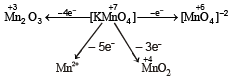QUESTION: 9

The radius of La3+ (Atomic number of La = 57) is 1.06Å.
Which one of the following given values will be closest to the radius of Lu3+ (Atomic number of Lu = 71) ?

Solution: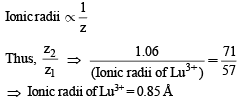QUESTION: 10

Ammonia forms the complex ion [Cu(NH3)4]2+ with copper ions in alkaline solutions but not in acidic solutions. What is the reason for it ?

Solution: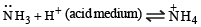QUESTION: 11

One mole of the complex compound Co(NH3)5Cl3, gives 3 moles of ions on dissolution in water. One mole of the same complex reacts with two moles of AgNO3 solution to yield two moles of AgCl (s). The structure of the complex is

Solution: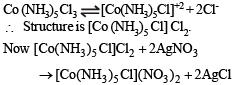QUESTION: 12

In the coordination compound, K4[Ni(CN)4], the oxidation state of nickel is

Solution:

Let the O. No of Ni in K4[Fe(CN)6] be = x then
4 (+ 1) + x + (–1) × 4 = 0  ⇒ 4 + x – 4 = 0 x = 0

QUESTION: 13

A red solid is insoluble in water. However it becomes soluble if some KI is added to water. Heating the red solid in a test tube results in liberation of some violet coloured fumes and droplets of a metal appear on the cooler parts of the test tube. The red solid is

Solution:

When KI is added to mercuric iodide it disssolve in it and form complex.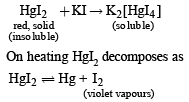QUESTION: 14

A reduction in atomic size with increase in atomic number is a characteristic of elements of

Solution:

f-block elements show a regular decrease in atomic size due to lanthanide/actinide contraction.

QUESTION: 15

What would happen when a solution of potassium chromate is treated with an excess of dilute nitric acid?

Solution:

When a solution of potassium chromate is treated with an excess of dilute nitric acid. Potassium dichromate and H2O are formed.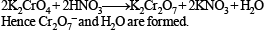QUESTION: 16

Which one of the following nitrates will leave behind a metal on strong heating ?

Solution:

AgNO3 on heating till red hot decomposes as follows :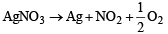QUESTION: 17

Of the following outer electronic configurations of atoms, the highest oxidation state is achieved by which one of them ?

Solution:

(n–1)d5ns2 attains the maximum O.S. of + 7

QUESTION: 18

The soldiers of Napolean army while at Alps during freezing winter suffered a serious problem as regards to the tin buttons of their uniforms. White metallic tin buttons got converted to grey power. This transformation is related to

Solution: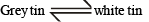Grey tin is brittle and crumbles down to powder in very cold climate The conversion of grey tin to white tin is acompained by increase in volume., This is knwon as tin plaque or tin disease.

QUESTION: 19

Among the properties (a) reducing (b) oxidising (c) complexing, the set of properties shown by CN ion towards metal species is

Solution:

CN ion acts good complexing a s well as reducing agent.

QUESTION: 20

The coordination number of a central metal atom in a complex is determined by

Solution:

The coor dination number of centr al metal atom in a complex is equal to number of monovalent ligands, twice the number of bidentate ligands and so on, around the metal ion bonded by coordinate bonds.
Hen ce coordin ation number = no. of s bonds for med by metals with ligands

QUESTION: 21

Which one of the following complexes is an outer orbital complex ?

(Atomic nos. : Mn = 25; Fe = 26; Co = 27, Ni = 28)

Solution:

Hybridisation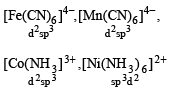Hence [ Ni(NH3)6]2+ is outer orbital complex.

QUESTION: 22

Coordination compounds have great importance in biological systems. In this context which of the following statements is incorrect ?

Solution:

The chlorophyll molecule plays an impor tant role in photosynthesis, contain porphyrin ring and the metal Mg not Ca.

QUESTION: 23

Cerium (Z = 58) is an important member of the lanthanoids. Which of the following statements about cerium is incorrect?

Solution:

The +4 oxidation state of cerium is also known in solution.

QUESTION: 24

Which one of the following has largest number of isomers ?

Solution:

Isomers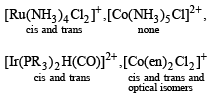QUESTION: 25

The correct order of magnetic moments (spin only values in B.M.) anong is

(Atomic nos. : Mn = 25, Fe = 26, Co = 27)

Solution: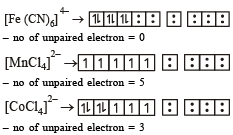NOTE :The greater the number of unpaired electrons, greater the magnitude of magnetic moment. Hence the correct order will be

[MnCl4] > [CoCl4]– – > [Fe(CN)6]4–

QUESTION: 26

The oxidation state Cr in [Cr (NH3)4 Cl2]+ is

Solution:

Oxidation state of Cr in [Cr (NH3)4 Cl2]+.
Let it be x,  1 × x + 4 × 0 + 2 × (–1) = 1 Therefore x = 3.

QUESTION: 27

Heating mixture of Cu2O and Cu2S will give

Solution:

2Cu2O + Cu2S → 6Cu + SO,
self reduction.

QUESTION: 28

The oxidation state of chromium in the final product formed by the reaction between Kl and acidified potassium dichromate solution is:

Solution: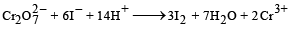oxidation state of Cr is 3+.

QUESTION: 29

Calomel (Hg2Cl2) on reaction with ammonium hydroxide gives

Solution:

Hg2Cl2 + 2NH4OH → HgNH2Cl +NH4Cl + 2H2O

QUESTION: 30

The lanthanide contraction is responsible for the fact that

Solution:

NOTE : In vertical columns of transition elements, there is an increase in size from first member to second member as expected but from second member to third member, there is very small chang in size and some times sizes are  same. This is due to lanthanide contraction this is the reason for Zr and Hf to have same radius.

QUESTION: 31

The IUPAC name of the coordination compound K3[Fe(CN)6] is

Solution:

K3[Fe(CN)6] is potassium hexacyano ferrate (III).

QUESTION: 32

Which of the following compounds shows optical isomerism?

Solution: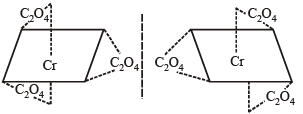Non-superimposable mirror images, hence optical isomers.

QUESTION: 33

Which one of the following cyano complexes would exhibit the lowest value of paramagnetic behaviour ?

(At. Nos : Cr = 24, Mn = 25, Fe = 26, Co = 27)

Solution: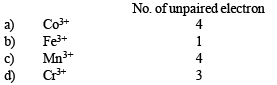The effective magnetic moment is given by the number of unpaired electrons in a substance, the lesser the number of unpaired electrons lower is its magnetic moment in Bohr – Magneton and lower shall be its paramagnetism

QUESTION: 34

The value of the ‘spin only’ magnetic moment for one of the following configurations is 2.84 BM. The correct one is

Solution:

d5 –––– strong ligand field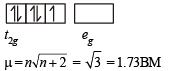d3–– in weak as well as in  strong field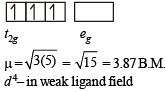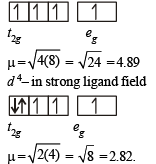QUESTION: 35

Which of the following factors may be regarded as the main cause of lanthanide contraction?

Solution:

In lanthanides, there is poorer shielding of 5 d electrons by 4f electrons resulting in greater attraction of the nucleus over 5d electrons and contraction of the atomic radii.

QUESTION: 36

The IUPAC name for the complex [Co(NO2)(NH3)5]Cl2 is:

Solution:

[Co(NO)2(NH3)5]Cl2

pentaammine nitrito-N-cobalt (III) chloride

QUESTION: 37

A metal, M forms chlorides in its +2 and +4 oxidation states.

Which of the following statements about these chlorides is correct?

Solution:

Metal atom in the lower oxidation state forms the ionic bond and in the higher oxidation state the covalent bond. because higher oxidation state means small size and great polarizing power and hence greater the covalent character. Hence MCl2 is more ionic than MCl4.

QUESTION: 38

Nickel (Z = 28) combines with a uninegative monodentate ligand X– to form a paramagnetic complex [NiX4]2–. The number of unpaired electron(s) in the nickel and geometry of this complex ion are, respectively :

Solution:

[NiX4]2–, the electronic configuration of Ni2+ is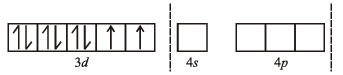It contains two unpaired electrons and the hybridisation is sp3 (tetrahedral).

QUESTION: 39

In Fe(CO)5, the Fe – C bond possesses

Solution:

Due to some backbonding by sidewise overlapping of between d-orbitals of metal and p-orbital of carbon, the Fe–C bond in Fe(CO)5 has both s and p character.

QUESTION: 40

Lanthanoid contraction is caused due to

Solution:

The configuration of Lanthanides show th at the additional electron enters the 4f subshell. The shielding of one 4 f electron by another is very little or imperfect.
The imperfect shielding of f electrons is due to the shape of f orbitals which is very much diffused. Thus as the atomic number increases, the nuclear charge increases by unity at each step. While no comparable increase  in the mutual shielding effect of 4f occurs.
This causes a contraction in the size of the 4 f subshell. as a result atomic and ionic radii decreases gradually from La to Lu.

QUESTION: 41

How many EDTA (ethylen ediaminetetraacetic acid) molecules are required to make an octahedral complex with a Ca2+ ion?

Solution:

EDTA has hexadentate four donor O atoms and 2 donor N atoms and for the formation of octahedral complex one molecule is required

QUESTION: 42

The "spin-only" magnetic moment [in units of Bohr magneton, (µB)] of Ni2+ in aqueous solution would be (At.
No. Ni  = 28)

Solution:

The number of unpaired electrons in Ni2+(aq) = 2
Water is weak ligand hence no pairing will take place spin magnetic moment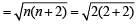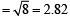QUESTION: 43

The stability of dihalides of Si, Ge, Sn and Pb increases steadily in the sequence

Solution:

Reluctance of valence shell electrons to participate in bonding is called inert pair effect. The stability of lower oxidation state (+2 for group 14 element) increases on going down the group. So the correct order is
SiX2 < GeX2 <  SnX2< PbX2

QUESTION: 44

Identify the incorrect statement among the following:

Solution:

4f orbital is nearer to nucleus as compared to 5f orbital therefore, shielding of 4f is more than 5f.

QUESTION: 45

Which of the following has a square planar geometry?

(At. nos.: Fe = 26, Co = 27, Ni = 28, Pt = 78)

Solution:

Complexes with dsp2 hybridisation are square planar.
So [PtCl4]2– is square planar in shape.

QUESTION: 46

The actinoids exhibit more number of oxidation states in general than the lanthanoids. This is because

Solution:

NOTE :  More the distance between nucleus and outer orbitals, lesser will be force of attraction on them.
Distance between nucleus and 5f orbitals is more as compared to distance between 4f orbital and nucleus.
So actinoids exhibit more number of oxidation states in general than the lanthanoids.

QUESTION: 47

The coordination number and the oxidation state of the element ‘E’ in the complex

[E (en)2 (C2O4)]NO2 (where (en) is ethylene diamine) are, respectively,

Solution:

In the given complex we have two bidentate ligands (i.e en and  C2O4), so coordination number of E is 6 (2 × 2 + 1 × 2 = 6)
Let the oxidation state of E in complex be x, then [x + (–2) = 1]  or   x – 2 = 1 or x = + 3, so its oxidation state is + 3
Thus option (d) is correct.

QUESTION: 48

Largern umber of oxidation states are exhibited by the actinoids than those by the lanthanoids, the main reason being

Solution:

NOTE :  The main reason for exhibiting larger number of oxidation states by actinoids as compared to lanthanoids is lesser energy difference between 5 f and 6d orbitals as compared to that between 4f and 5d orbitals.
In case of actinoids we can remove electrons from 5f as also from 6 d and due to this actinoids exhibit larger number of oxidation state than lanthanoids. Thus the correct answer is option (b)

QUESTION: 49

In which of the following complexes of the Co (at. no. 27), will the magnitude of Δ° be the hightest?

Solution:

In octahedral complex the magnitude of Δ° will be highest in a complex having strongest ligand. Of the given ligands CN is strongest so Δ° will be highest for (Co(CN)6)3–. Thus option (a) is correct.

QUESTION: 50

Amount of oxalic acid present in a solution can be determined by its titration with KMnO4 solution in the presence of H2SO4.The titration gives unsatisfactory result when carried out in the presence of HCl, because HCl

Solution:

The titration of oxalic acid with KMnO4 in presence of HCl gives unsatisfactory result because of the fact that KMnO4 can also oxidise HCl along with oxalic acid.
HCl on oxidation gives Cl2 and HCl reduces KMnO4 to Mn2+ thus the correct answer is (c).

QUESTION: 51

Which of the following has an optical isomer

Solution: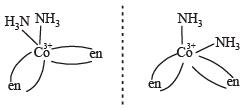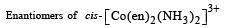QUESTION: 52

In context with the transition elements, which of the following statements is incorrect?

Solution:

Lower oxidation state of an element forms more basic oxide and hydroxide, while the higher oxidation state will form more acidic oxide/hydroxide. For example,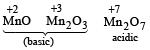QUESTION: 53

Which of the following pairs represent linkage isomers?

Solution:

The SCN  ion can coordinate through S or N atom giving rise to linkage isomerism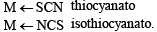QUESTION: 54

Knowing that the chemistry of lanthanoids(Ln) is dominated by its + 3 oxidation state, which of the following statements is incorrect?

Solution:

Most of the Ln3+ compounds except La3+ and Lu3+ are coloured due to the presence of f-electrons.

QUESTION: 55

A solution containing 2.675 g of CoCl3. 6NH3 (molar mass = 267.5 g mol–1) is passed through a cation exchanger. The chloride ions obtained in solution were treated with excess of AgNO3 to give 4.78 g of AgCl (molar mass = 143.5 g mol–1). The formula of the complex is (At. mass of Ag = 108 u)

Solution: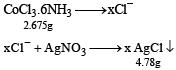Number of moles of the complex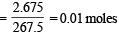Number of moles of AgCl obtained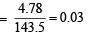moles
∴ No. of moles of AgCl obtained = 3 × No. of moles of complex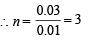QUESTION: 56

Which one of the following has an optical isomer?

Solution:

For a substan ce to be optical isomer followin g conditions should be fulfiled (a)  A coordination compound which can rotate the plane of polarised light is said to be optically active. (b) When the coordination compounds have same formula but differ in their abilities to rotate directions of the plane of polarised light are said to exhibit optical isomerism and the molecules are optical isomers. The optical isomers are pair of molecules which are nonsuperimposable mirror images of each  other. (c) This is due  to the absence of elements of symmetry in the complex. (d) Optical isomerism is expected in tetrahedral complexes of the type Mabcd.
Based on this only option (2) shows optical isomerism [Co(en)3]3+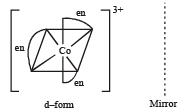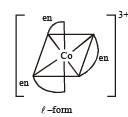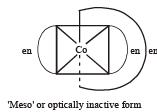Complexes of Zn++ cannot show optical isomerism as they are tetrahedral complexes with plane of symmetry.
[Co(H2O)4(en)]3+ have two planes of symmetry hence it is also optically inactive.
Hence the formula of the complex is [Co(NH3)6]Cl3

QUESTION: 57

Which of the following facts about the complex [Cr(NH3)6]Cl3 is wrong?

Solution:

[Cr(NH3)6]Cl3 is an inner orbital complex, because in this complex d-orbital used is of lower quantum number ie (n – 1). It results from d2 sp3 (inner orbital) hybridization.

QUESTION: 58

In context of the lan thanoids, which of the followin g statements is not correct?

Solution:

The most stable oxidation states of lanthanides is + 3.

QUESTION: 59

The magnetic moment (spin only) of [NiCl4]2– is :

Solution: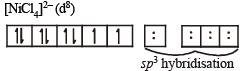i.e the number of unpaired electrons in [NiCl4]2– is 2.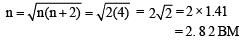QUESTION: 60

The outer electron configuration of Gd (Atomic No. : 64) is :

Solution:

The configuration of Gd is 4f7 5d1 6s2.

QUESTION: 61

Which among the following will be named as dibromidobis (ethylene diamine) chromium (III) bromide?

Solution:

[Cr(en)2Br2]Br

dibromidobis (ethylenediamine) chromium (III) Bromide.

QUESTION: 62

Iron exhibits +2 and +3 oxidation states. Which of the following statements about iron is incorrect ?

Solution:

Fe3+ is easily h ydrolysed than Fe2+ due to mor e positive charge.

QUESTION: 63

Which of the following complex species is not expected to exhibit optical isomerism ?

Solution:

Octahedral coordination entities of the type Ma3b3 exhibit Geometrical isomerism. The compound exists both as facial and meridional isomers.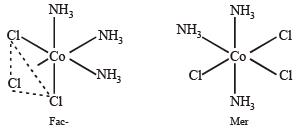QUESTION: 64

Which of the following arrangements does not represent the correct order of the property stated against it ?

Solution: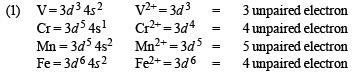hence the correct order of paramagnetic behaviour

V2+ < Cr2+ = Fe2+ < Mn2+

(2) For the same oxidation state, the ionic radii generally decreases as the atomic number increases in a particular transition series. hence the  order is Mn++ > Fe++ > Co++ > Ni++

(3) In solution, the stability of the compounds depends upon electrode potentials, SEP of the transitions metal ions are given as

Co3+ / Co = + 1.97, Fe3+ / Fe = + 0.77 ; Cr3+ / Cr2+ = – 0.41, Sc3+ is highly stable as it does not show + 2 O. S.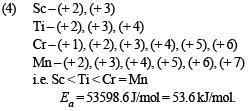QUESTION: 65

Four successive members of the first row transition elements are listed below with atomic numbers. Which one of them is expected to have the highest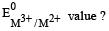Solution: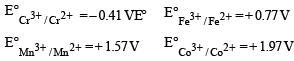QUESTION: 66

The octah edral complex of a metal ion M3+ with four monodentate ligands L1, L2, L3 and L4 absorb wavelengths in the region of red, green, yellow and blue, respectively. The increasing order of ligand strength of the four ligands is:

Solution: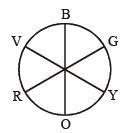For a given metal ion, weak field ligands create a complex with smaller Δ, which will absorbs light of longer l and thus lower frequency. Conservely, stronger field ligands create a larger Δ, absorb light of shorter l and thus higher v i.e. higher energy.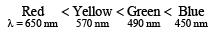So order of ligand strength is

L1 < L3 < L< L4

QUESTION: 67

Which series of reactions correctly represents chemical reactions related to iron and its compound?

Solution:

In equation (i) Fe2(SO4)3 and in equation (ii) Fe2(SO4)3 on decomposing will form oxide instead of Fe.
The correct sequence of reactions is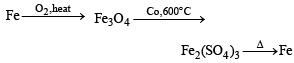QUESTION: 68

Which of the following compounds is not colored yellow ?

Solution:
QUESTION: 69

Match the catalysts to the correct processes :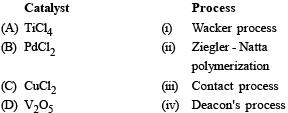Solution:

(A) - (ii), (B) - (i), (C) - (iv), (D) - (iii)

QUESTION: 70

The number of geometric isomers that can exist for square planar complex [Pt (Cl) (py) (NH3) (NH2OH)]+ is

(py = pyridine) :

Solution:

Square planar complexes of type M[ABCD] form three isomers. Their position may be obtained by fixing the position of one ligand and placing at the trans position any one of the remaining three ligands one by one.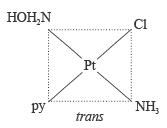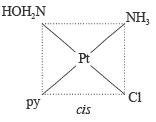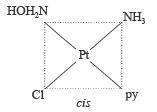QUESTION: 71

The color of KMnO4 is due to :

Solution:

L → M charge transfer spectra. KMnO4 is colored because it absorbs light in the visible range of electromagnetic radiation. The permanganate ion is the source of color, as a ligand to metal, (L → M) charge transfer takes place between oxygen's p orbitals and the empty d-orbitals on the metal. This charge transfer takes place when a photon of light is absorbed, which leads to the purple color of the compound.

QUESTION: 72

Which of the following compounds is metallic and ferromagnetic?

Solution:

Out of all the four given metallic oxides CrO2 is attracted by magnetic field very  strongly. The effect persists even when the magnetic field is removed. Thus CrO2 is metallic and ferromagnetic in nature.

QUESTION: 73

Which one of the following complexes shows optical isomerism?

(en = ethylenediamine)

Solution:

Optical isomerism occurs when a molecule is non-superimposable with its mirror image hence the complex cis-[Co(en)2Cl2]Cl is optically active.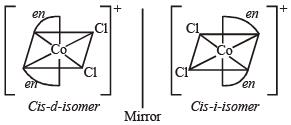QUESTION: 74

The pair having the same magnetic moment is:

[At. No.: Cr = 24, Mn = 25, Fe = 26, Co = 27]

Solution: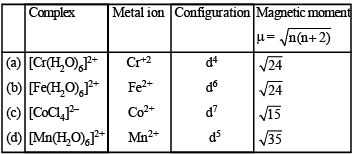Since (a) and (b), each has 4 unpaired  electrons, they will have same magnetic moment.